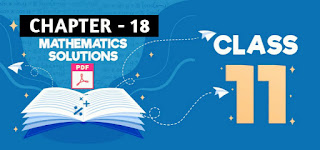# Antiderivatives Class 11 Mathematics Solutions | Exercise - 18.5## Chapter - 18  Antiderivatives

This year, in class 11, students are introduced to antiderivatives, which are a type of calculus. Antiderivatives can be used to calculate the area under a function, evaluate an indefinite integral, and solve differential equations. Through the use of integration by substitution, integration by parts, and trigonometric substitution, students learn how to calculate antiderivatives.

Also, students are introduced to the Fundamental Theorem of Calculus so that they will be able to calculate antiderivatives. Furthermore, they are taught how to identify a function's antiderivative so that they can understand how to evaluate definite integrals using the substitution rule.

Let us first take a brief look at the concept of antiderivaties and the subtopics associated with antiderivatives, in addition to some of the most important formulas and equations involved in the solution side of things.

### Introduction

Antiderivatives, also known as indefinite integrals, are the inverse operation of derivatives. In other words, an antiderivative is the original function from which a derivative was derived.

For example, the derivative of f(x) = x² is f'(x) = 2x. Therefore, the antiderivative of 2x is f(x) = x². Antiderivatives can be used to find the area under a curve, and can also be used to solve differential equations.

### Definite Integrals

Definite integral is a mathematical term used to denote a specific type of antiderivative. It is a tool used to calculate the area bounded by a curve, the volume of a solid of revolution, the length of a curve, and other such related quantities. It is a definite limit of a sum, known as a Riemann sum and denoted by an integral sign. It is defined as the limit of the sum of the products of the function values and the lengths of the subintervals of a partition of a given interval.

### Exercise - 18.5

This PDF contains all handwritten notes of class 11 Antiderivatives chapter, including solutions of Exercise 18.5. If you want the solutions of other exercises, click the button above.

NoteScroll the PDF to view all Solution

You are not allowed to post this PDF in any website or social platform without permission.

## Is Class 11 Mathematics Guide Helpful For Student ?

I have published this Notes for helping students who can't solve difficult maths problems. Student should not fully depend on this note for completing all the exercises. If you totally depend on this note and simply copy as it is then it may affect your study.

Student should also use their own will power and try to solve problems themselves. You can use this mathematics guide PDF as a reference. You should check all the answers before copying because all the answers may not be correct. There may be some minor mistakes in the note, please consider those mistakes.

## How to secure good marks in Mathematics ?

As, you may know I'm also a student. Being a student is not so easy. You have to study different subjects simultaneously. From my point of view most of the student are weak in mathematics. You can take me as an example, I am also weak in mathematics. I also face problems while solving mathematics questions .

If you want to secure good marks in mathematics then you should practise them everyday. You should once revise all the exercise which are already taught in class. When you are solving maths problems, start from easy questions that you know already. If you do so then you won't get bored.

Maths is not only about practising, especially in grade 11 you to have the basic concept of the problem. When you get the main concept of the problem then you can easily any problems in which similar concept are applied.

When your teacher tries to make the concept clear by giving examples then all students tries to remember the same example but you should never do that. You can create your own formula which you won't forget later.

If you give proper time for your practise with proper technique then you can definitely score a good marks in your examination.

Disclaimer: This website is made for educational purposes. If you find any content that belongs to you then contact us through the contact form. We will remove that content from our as soon as possible.

If you have any queries then feel free to comment down.
•